# Hexagons are the Bestagons (JS edition) · Part one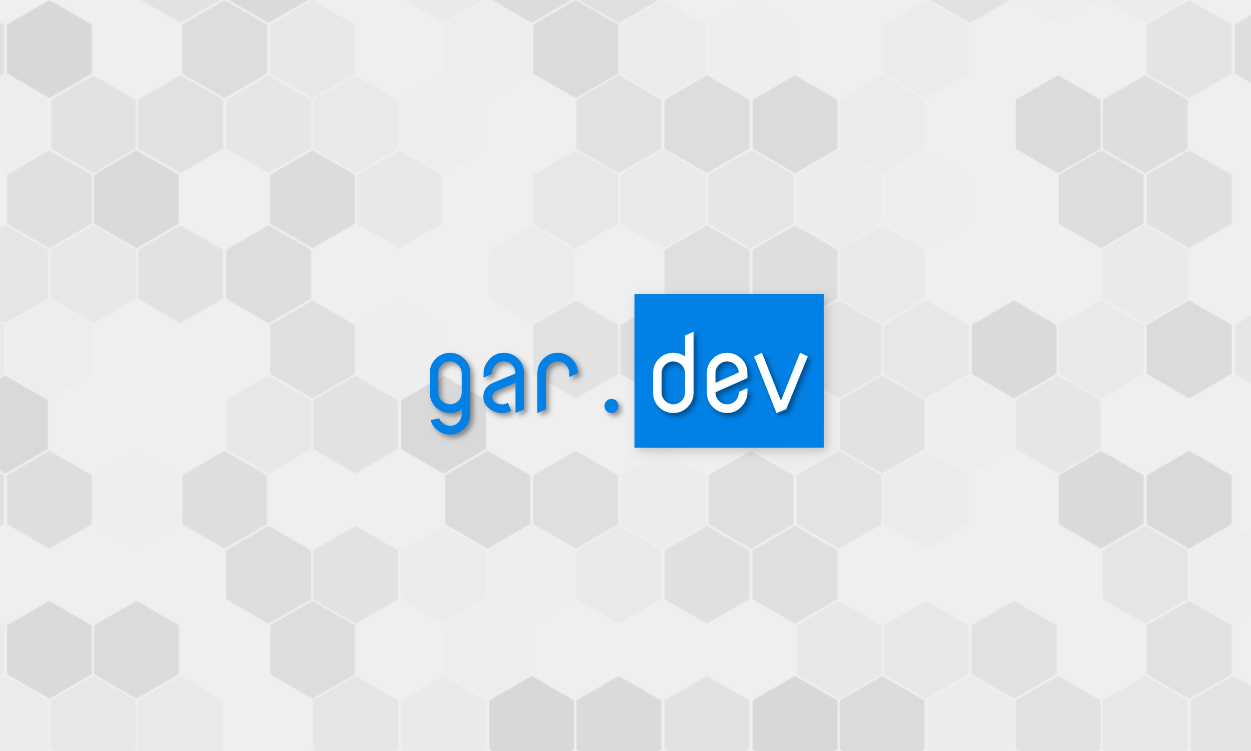As we all know, Hexagons are the Bestagons - we see them in honeycombs, they are in snowflakes and pencils, there’s one on Saturn, and they are... (math incoming) the only regular polygon to tile a plane without resorting to debasing self-divison 😅.

When I started rebuilding my website, I knew I wanted a live, configurable background and it quickly became clear it’ll be rendered on a canvas. I was playing around, drawing various 2D shapes, when I remembered the CGP Grey video - hexagons... The Bestagons were the obvious choice!

In this series of tutorials I’ll show you how I built this background.

## Part one: how to render a hexagon?

OK, so how do we render a regular hexagon? We want to draw 6 straight lines of equal length. To form a hexagon, the angle between every two sides has to be 120°.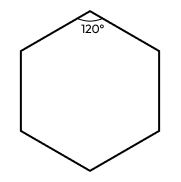Let’s explore our options for drawing stuff on a canvas. Here's some API methods from CanvasRenderingContext2D, the interface from the Canvas API that we use to draw in two dimensions:

• arc() - creates a circular arc. Hexagons only have straight walls, `arc` is definitely not our thing;
• ellipse() - creates an ellipse. Same as above, cool, but not for us;
• rect() - adds a rectangle to the current path. Finally, some straight lines. Still won’t work for us though - rectangles are obviously the inferior choice... and we can't really draw a hex using a full reactangle;
• lineTo() - adds a straight line to the current sub-path. This is our thing!

Unfortunately, there doesn’t seem to be anything more convenient in the API. No `fillHex()` function yet 🙄. We’ll have to draw our hexagons line by line.

So, let’s take a look at the `lineTo()` method - it accepts an `x` and a `y` coordinate. Here’s the example from MDN:

``````const canvas = document.getElementById("canvas");
const ctx = canvas.getContext("2d");

ctx.beginPath(); // Start a new path
ctx.moveTo(30, 50); // Move the pen to (30, 50)
ctx.lineTo(150, 100); // Draw a line to (150, 100)
ctx.stroke(); // Render the path
``````

OK, so in order to draw a hex, we need to figure out the coordinates of all its vertices.

## Computing the vertices

Let’s give the vertices of our future Hex names - A, B, C, D, E, F: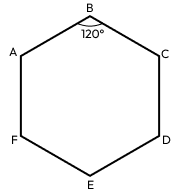And let’s say we start from vertex A. Our code will then look something like this:

``````const canvas = document.getElementById("canvas");
const ctx = canvas.getContext("2d");

ctx.beginPath(); // Start a new path
ctx.moveTo(vertexA.x, vertexA.y); // move the pen to vertex A
ctx.lineTo(vertexB.x, vertexB.y); // draw a line from A to B
ctx.lineTo(vertexC.x, vertexC.y); // draw a line from B to C
ctx.lineTo(vertexD.x, vertexD.y); // draw a line from C to D
ctx.lineTo(vertexE.x, vertexE.y); // draw a line from D to E
ctx.lineTo(vertexF.x, vertexF.y); // draw a line from E to F
ctx.lineTo(vertexA.x, vertexA.y); // ...and finally, from F to A to finish the hex
ctx.closePath();
ctx.stroke(); // Render the path
``````

The next question we have to answer is - if vertex A has coordinates `(0, 0)`, where is vertex B?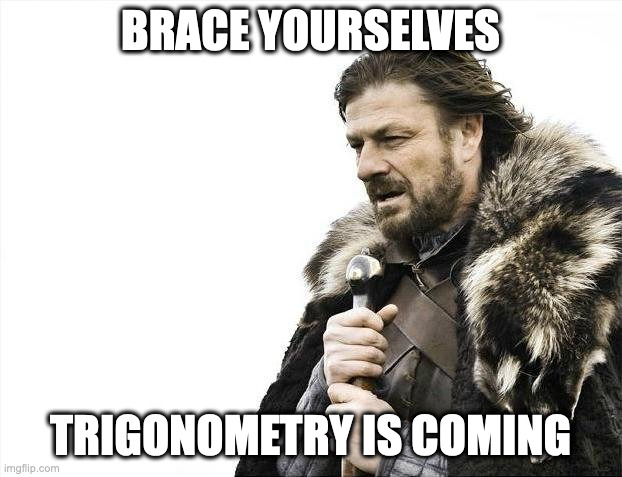First, we need to define the length of our hex’s side. Let’s hardcode it to `10px`. Then, figure out where B is.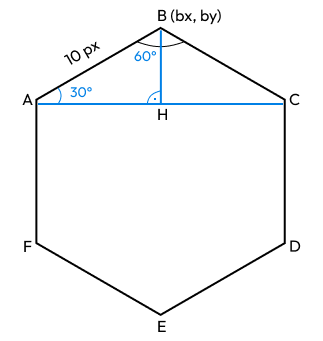Let’s call the coordinates of A `(ax, by)` , and of B `(bx, by)` . We draw a line between A and C and the height of the ABC triangle - BH.

We can now see that:

• `bx == ax + length(AH)` - B is straight on top of H, so `bx` is the same as the `x` coordinate of H (`hx`); H is `length(AH)` away from A, so `hx` is `ax + length(AH)`
• `by == ay - length(BH)` - B is `length(BH)` away from H, so `by = hy - length(BH)`; and because A and H are on the same `y``hy` is the same as `ay`.

If we find the lengths of AH and BH, we’re good to go. To find them, we need a bit of math “magic”:

• `∠AHB` is 90°. `∠ABH` is half of `∠ABC`, so it’s 60°, therefore `∠HAB` is 180 - (90 + 60) = 30°.
• From trigonometry, we know that `length(AH) = cos(∠HAB) * length(AB)`, and `length(BH) = sin(∠AHB) * length(AB)`

Let's call the length of AB `hexSide`, then:

• `length(AH) = cos(30°) * hexSide`
• `length(BH) = sin(30°) * hexSide`

## Math over!

We’re mostly done with the math. Back to code!

``````const COS_30 = Math.cos(Math.PI / 6);
const SIN_30 = Math.sin(Math.PI / 6);

const hexSide: number = 10; // the side of our hex is hardcoded to 10px

// Note: in real life, lacking the diagram above, `AH` means nothing.
// I would keep this as `COS_30 * hexSide` or try to give a more
// meaningful name.
const AH = COS_30 * hexSide;
const BH = SIN_30 * hexSide;

const vertexA = { x: 0, y: 0 };
const vertexB = { x: vertexA.x + AH, y: vertexA.y - BH };
``````

And we have our vertex B!

At this point I suggest you pause for a bit and try to figure out the coordinates of C, D, E, and F. Use what we already know - the coordinates of A and B, `hexSide`, `AH`, and `BH`.

Here’s the solution:

``````const vertexA = { x: 0, y: 0 };
const vertexB = { x: vertexA.x + AH, y: vertexA.y - BH };
const vertexC = { x: vertexB.x + AH, y: vertexA.y };
const vertexD = { x: vertexC.x, y: vertexC.y + hexSide };
const vertexE = { x: vertexB.x, y: vertexD.y + BH };
const vertexF = { x: vertexA.x, y: vertexD.y };
``````

So, now that we have all vertex coordinates, we're finally ready to render our hexagon:

``````<html>
<body>
<canvas id="canvas"></canvas>
<script>
const COS_30 = Math.cos(Math.PI / 6);
const SIN_30 = Math.sin(Math.PI / 6);

// Coords of our first vertex.
const start = { x: 100, y: 100 };

// Length of our hex's side.
const hexSide = 100;

// Find or create a canvas.
const canvas = document.querySelector('#canvas');
canvas.width = 400;
canvas.height = 400;

const ctx = canvas.getContext('2d');

// Compute the vertices (same as above).
const vertexA = start;
const vertexB = {
x: vertexA.x + hexSide * COS_30,
y: vertexA.y - hexSide * SIN_30
};
const vertexC = { x: vertexB.x + hexSide * COS_30, y: vertexA.y };
const vertexD = { x: vertexC.x, y: vertexC.y + hexSide };
const vertexE = { x: vertexB.x, y: vertexD.y + hexSide * SIN_30 };
const vertexF = { x: vertexA.x, y: vertexA.y + hexSide };

// List all vertices. Remember - we have to `lineTo()` to vertex A to
// finish the hex.
const vertices = [
vertexA, vertexB, vertexC,
vertexD, vertexE, vertexF, vertexA
];

// Begin a new sub-path at vertex0.
ctx.moveTo(vertices.x, vertices.y);
ctx.beginPath();

// Add line to the sub-path from each vertex to the next one.
vertices.forEach(({ x, y }) => ctx.lineTo(x, y));

// End the sub-path.
ctx.closePath();

// Set the stroke color for our hex.
ctx.strokeStyle = 'rgb(0, 0, 0)';

// And finally, our Bestagon:
ctx.stroke();
</script>
</body>
</html>

``````

And the outcome is: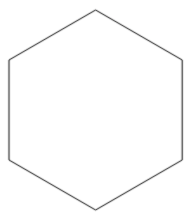This is it for Part one! Thank you for reading!

In Part two we will continue with rendering multiple hexes on a grid. Stay tuned 😉